# Complex Numbers in Google Sheets

Complex numbers are numbers in the form a + bi, where i is the square root of -1.

What on earth does that mean?

## Imaginary And Complex Numbers

To start, consider an integer, say the number 4. The square root of 4 is 2. Easy peasy.

Now consider -4. What’s the square root of that?

It’s not -2, because -2 * -2 = 4 (a minus multiplied by a minus is a positive in mathematics).

No real number will equal the square root of – 4, so we need a new number.

This new number is called an imaginary number (no, I didn’t just make that up!) and is denoted by i and defined as the square root of -1.

`i = √-1`

or put another way:

`i² = -1`

A complex number is a number that has real and imaginary parts, and can be expressed in the form:

`a + bi`

where a and b are real numbers and i is the imaginary number defined above.

We can look at complex numbers visually in a 2-dimensional plane, with the x-axis representing the real numbers and the y-axis representing the imaginary numbers: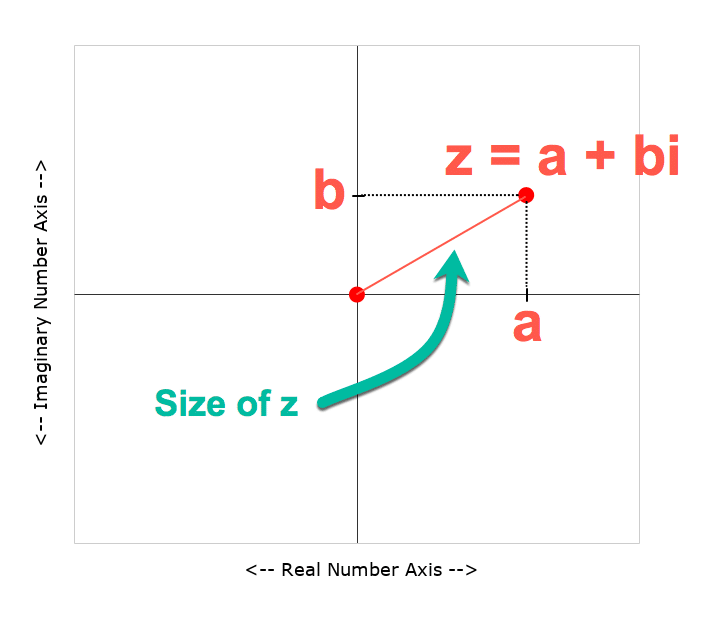Complex numbers are usually represented by the letter z, and written:

`z = a + bi`

Let’s go and create one in Google Sheets!

## Complex Numbers In Google Sheets

Let’s create a complex number in Google Sheets.

Starting in a blank Sheet, add the numbers 3 and 2 into cells A1 and B1 respectively.

In C1, add this formula with the COMPLEX function:

`=COMPLEX(A1,B1,"i")`

This is what our complex number looks like in Google Sheets: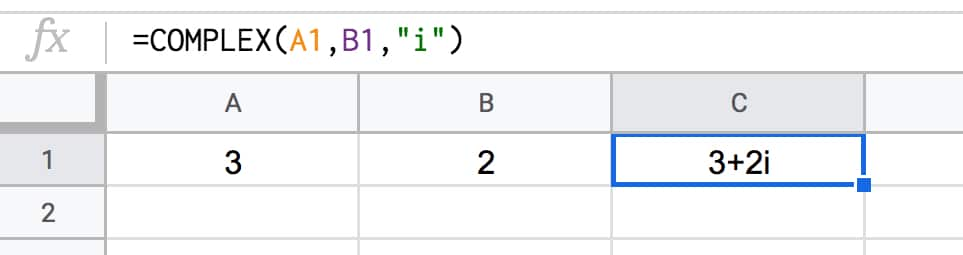### Size Of A Complex Number

We can calculate the size of a complex number, called its absolute value or modulus value and written |z|, by measuring how far it is from the center point (0,0).

We do this using the Pythagorean formula for the longest side of a right angle triangle, namely the square root of the squares of the other two sides.

Google Sheets has a handy function, called IMABS, that will calculate this value for us.

Put this formula into cell D1:

`=IMABS(C1)`

It gives the result 3.605551275.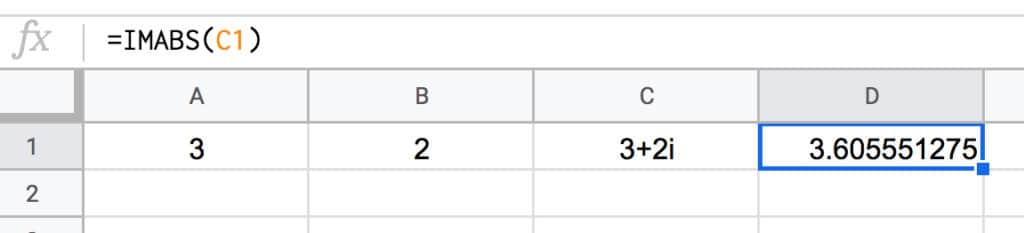This is the same as doing the square root of the squares of the other two sides:

`=SQRT(3^2+2^2)`

The next operation we need to know how to do with complex numbers is how to add them.

What’s 3 + 2i added to 5 + 7i?

To get the answer we add the real parts and imaginary parts separately, and combine into a new complex number.

So

```z = (3 + 2i) + (2 + 7i) = (3 + 2) + (2i + 7i) = 5 + 9i```

Back in our Google Sheet, I’ve added a new row for the new complex number and used the IMSUM function to add them together:

`=IMSUM(C1:C2)`

which looks like this in our Google Sheet: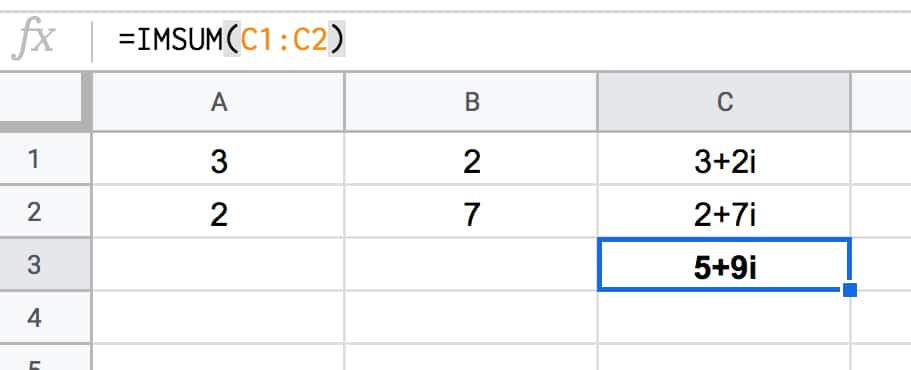### Squaring Complex Numbers

Another operation we need to perform on our complex numbers is to square them.

The IMPOWER function does the trick. It returns a complex number raised to a power.

In our Google Sheet, use this formula in cell D1:

`=IMPOWER(C1,2)`

which gives the answer 5 + 12i, calculated as follows:

```(3 + 2i)² = (3 + 2i) * (3 + 2i) = 3 * 3 + 2i * 3 + 3 * 2i + 2i * 2i = 9 + 6i + 6i + 4 * i² = 9 + 12i + 4 * -1 = 9 - 4 + 12i = 5 + 12i```

### Extracting Real And Imaginary Parts

You can use the IMREAL function to return the real coefficient of a complex number (the “a” part).

And IMAGINARY function returns the imaginary coefficient (the “b” part).

There are other, more specialized complex numbers functions in Google Sheets too, but they’re beyond the scope of this article.

## Why Are Complex Numbers Useful?

For mathematicians, complex numbers are just as “real” as real numbers.

They have applications in many areas of mathematics, physics, and engineering.

One area where you may have seen them without realizing, is in fractal geometry and specifically pictures of a beautiful set of complex numbers called the Mandelbrot set: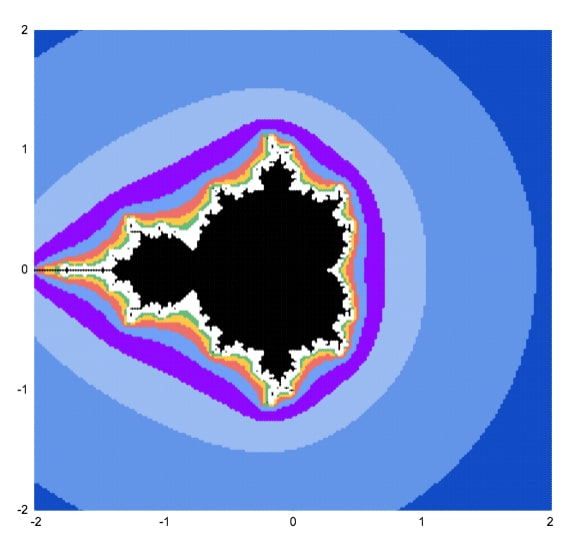## 3 thoughts on “Complex Numbers in Google Sheets”

1.Adam Beherns says:

Thanks! Too bad that function “Complex” creates a text string and not an actual complex number. Would be super helpful in my engineering if I can take say (220KV)^2 / (4+9j), which is a simple form of power equals volts squared over impedance, which in our case is complex due to resistance and reactance in we find in ever real transmission line. (yes we use “j’s” and not “i’s” in electrical engineering…

1.Ben says:

2.Jody says: